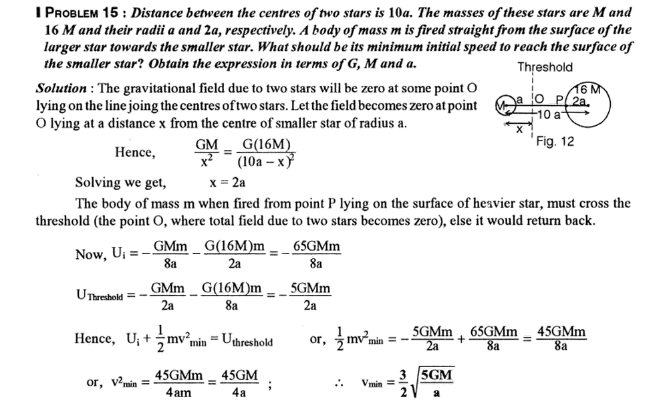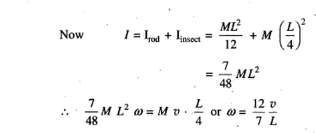# Gravitation

Q1. The distance between the centres of two Stars is 10a.  The masses of these stars are M and 16 M and their radii a and 2a respectively. A body of mass m is fired straight from the surface of the larger Star towards the smaller Star. What should be its minimum initial speed to reach the surface of the smaller star? Obtain the expression in terms of G, M and a.Q2. A homogeneous rod AB of length L and Mass M is pivoted at the centre O in such a way that it can rotate freely in the vertical plane. The rod is initially in the horizontal position. An insect S of the same mass M falls vertically with speed v on the point C midway between O and B. Determine the initial angular velocity ω in terms of v and L.1.  If the radius of the earth shrinks by 2.0%, mass remaining constant, then how would the value of acceleration due to gravity change?  [Value of g increases by 4%]

2. At what height from the surface of the earth, will the value of g be reduced by 36% from the value at the surface? The radius of the earth = 6400 Km [1600 km]

3. At what height above the earth’s surface, the value of g is half of its value on the earth’s surface? Given its radius is 6400 Km. [Root(2)-1]R

4. Find the percentage decrease in the weight of a body when taken to a height of 32 km above the surface of the earth. The radius of the earth is 6400 km. [1%]

5. What is the relation between h and depth d for the same change in g?
[d=2h] Hence the acceleration due to gravity at a height h above the earth’s surface will be the same as that at the depth d=2h below the surface of the earth. But this is valid when h<<R.

6. At what height above the surface of the earth, the value of g is same as in a mine 80 km deep? [40 km]

7. How much below the surface of the earth does the acceleration due to gravity i) reduces to 36% ii) reduces by 36% of its value on the surface of the earth? The radius of earth =6400 km. [i) 4096 km ii) 2304 km]

8. Draw a graph showing the variation of acceleration due to gravity g with distance r from the centre of the earth.